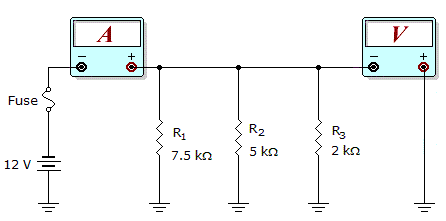# Electronics - Parallel Circuits - Discussion

### Discussion :: Parallel Circuits - General Questions (Q.No.11)

11.What would these meter readings indicate about the circuit in the given circuit?

Meter Readings: I = 7.6 mA, V = 12 V

 [A]. R1 is open. [B]. R2 is open. [C]. The fuse is open. [D]. The circuit is operating normally.

Explanation:

No answer description available for this question.

 Himanshu Verma said: (Nov 22, 2010) Case1 :When R1 is open, then the effective resistance R = R2||R3 i.e. 5K||2K = 10/7 K then current I = V/R = (12*7)/10K = 8.4 mA(Which is not correct ans i.e 7.6 mA) Case2 : When R2 is open, then the effective resistance R = R1||R3 i.e. 7.5K||2K = 30/19 K then current I = V/R = (12*19)/30K = 7.6 mA(Which is d correct ans i.e 7.6 mA) Case3: When the fuse is open, then it is not possible to obtain voltage of 12 V So the correct answer is option [b].

 Saichaitanya said: (Apr 3, 2011) CONSIDER THE FOLLOWING: In Parallel circuit, the current will differ and the voltage will remain same across all the branches. In other words, the voltage across R1, R2 and R3 will be 12V each. I1= 12/7.5K => 1.6mA I2= 12/5K => 2.4mA I3= 12/2K => 6.0mA In above, the total current 7.6mA will take place correctly when only I1 and I3 present *(since, I1 + I3 = 7.6mA). Hence, R2 must be discarded/ remove from the circuit. In other words, R2 is open.

 Mohan said: (Jun 24, 2011) I agree with Saichaithanya.

 Sudhansu said: (Oct 28, 2011) Well done Saichaitanya and Himanshu Verma.

 Shinoj said: (Jan 13, 2012) @ Saichaitanya, Perfect explanation.

 Sriram Pn said: (Mar 8, 2012) Saichaitanya perfect explanation

 Suthan said: (Aug 22, 2012) Fantastic Himanshu Verma & Saichaitanya ..Great work.

 Gigi said: (Feb 19, 2013) Do the total resistance in the cut by V/I that is 1.578. Then do the total combination or resist ivory in parallel which resisters combination gives 1.578 ohms and other resistor is open.

 Santosh said: (Sep 16, 2014) Voltmeter is in parallel connection, so reading is 12V. Now for resistance calculation which is connected parallel with 2k ohm resistance (R3): Equivalent resis. R = 12V/7.6mA = (30/19)K ohm. So, Rx ll R3 = (30/19)k. or, (1/Rx)+ (1+R3) = 1/(30/19)k. or, (1/Rx)+ (1/2) = (19/30). or, (1/Rx) = (19/30)-(1/2). or, 1/Rx = 4/30. or, Rx = 30/4 = 7.5k ohm. i.e., shows R1 is connected & R2 is opened.

 Pre said: (Jun 15, 2016) Well done @ Himanshu Verma.

 Samir said: (Jun 25, 2016) Thank you guys for giving a clear explanation.

 Addy said: (Jul 29, 2016) Just look at the fig. above, there is showing batteries positive lead is connected to ground. Not a short to another component which is also grounded. If positive supply connect to the ground else is there current flow in the circuit! Please tell me.

 Pratiksha said: (Mar 23, 2017) Well said, thanks for the given explanation.

 Maan said: (Jun 16, 2017) I agree @Saichaitanya.

 Saish said: (Aug 3, 2017) Yes, you are correct. Agree @Addy.

 Anomie said: (Apr 29, 2019) I=7.6mA so. ~7.6=12V/(7.5K*2K/9.5K). So R2 is not considered for the meter current so it can a open R2.